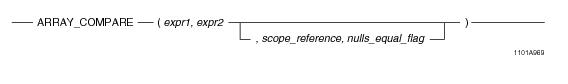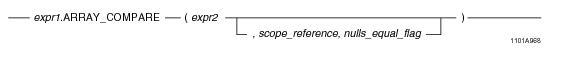## SQL Functions, Operators, Expressions, and Predicates

Product
Release Number
15.00
Language
English (United States)
Last Update
2018-09-24
dita:id
B035-1145
lifecycle
previous
Product Category

## ARRAY_COMPARE

### Purpose

Performs a pairwise comparison of the elements of two arrays and returns a value of 1, 0, or NULL to indicate whether or not the two array arguments contain the same element values.

### Syntax

System function syntax:Method-style syntax:where:

 Syntax element… Specifies… expr1 an ARRAY expression, which is one of the following: A reference to an ARRAY column An ARRAY constructor expression. See “ARRAY Constructor Expression” on page 195. A UDF expression A UDM expression expr2 an ARRAY expression, which is one of the following: A reference to an ARRAY column An ARRAY constructor expression. See “ARRAY Constructor Expression” on page 195. A UDF expression A UDM expression scope_reference an optional ARRAY scope reference. See “ARRAY Scope Reference” on page 198. nulls_equal_flag an optional flag used to specify how NULL element values should be compared. Valid values for this parameter are 0 and 1.

### ANSI Compliance

This is a Teradata extension to the ANSI SQL:2011 standard.

The function does a pairwise comparison of the elements of two arrays, following the rules for ARRAY comparison according to the ANSI standard.

### Usage Notes

The ARRAY_COMPARE function does a pairwise comparison of the two array arguments, and returns a scalar value indicating whether or not the two array expressions contain all of the same element values.

ARRAY_COMPARE follows the rules for ANSI array comparison as follows:

1 If either expr1 or expr2 evaluates to NULL, the result is NULL.

2 expr1 and expr2 are equal if and only if all the element values of expr1 are equal to the element values of expr2.

3 An element value of expr1 is equal to an element value of expr2 if and only if they both have the same value and both have the same position in the array.

4 If any non-NULL elements do not match for a given element of Array A and Array B, then this is considered a mismatch and ARRAY_COMPARE returns a value of 0.

5 If either or both values are NULL for a given element, and all other elements in the arrays match, this is considered as a mismatch according to the ANSI standard. The result in this case, according to the ANSI standard, is UNKNOWN. In Teradata, this evaluates to a NULL result.

If rule (1) or (5) above is satisfied, ARRAY_COMPARE returns NULL. If rules (2) and (3) are satisfied, ARRAY_COMPARE returns 1. Otherwise, ARRAY_COMPARE returns 0.

When the optional nulls_equal_flag is set to 1, then when both values are NULL for a given element, this is considered as a match, which is a deviation from the ANSI standard. If all other elements match, then rule (2) above applies.

If you specify the optional argument scope_reference, then the function is applied only to the elements within the limits of the given scope.

If an array argument contains any elements that are in an uninitialized state, an error is returned. Use a scope reference to avoid referencing a range of the ARRAY that contains uninitialized elements, or set any uninitialized elements to NULL. You can do this with the OEXTEND method. See “OEXTEND” on page 272.

### Restrictions

You cannot make relational comparisons of ARRAY values. You cannot use any of the relational comparison operators on ARRAY data. You can only make relational comparisons on individual elements of an ARRAY.

### Examples

In this example, the inventory table contains two ARRAY columns, sale_price_june and sale_price_july, which contain the following element values:

`sale_price_june[1:10] = 50, 100, 200, 90, 250, 550, 200, 200, 50, 75`
`sale_price_july[1:10] = 50, 100, 200, 90, 250, 550, 200, 200, 50, 75`

The output of the following query is 1 since all of the element values in the sale_price_june array are equal to the element values in the sale_price_july array.

`SELECT ARRAY_COMPARE(sale_price_june, sale_price_july)`
`FROM inventory;`

The following is the same query using method-style syntax.

`SELECT sale_price_june.ARRAY_COMPARE(sale_price_july)`
`FROM inventory;`

Consider the same arrays but with different element values as follows:

`sale_price_june[1:10] = 50, 100, 200, 90, 250, 550, 200, 200, 50, 75`
`sale_price_july[1:10] = 50, 100, 200, 90, 300, 550, 200, 200, 50, 75`

The output of the following query is 0 since the element value in position 5 differs in the two arrays.

`SELECT ARRAY_COMPARE(sale_price_june, sale_price_july)`
`FROM inventory;`

The following query compares the values of elements in positions 6 through 10. The output of this query is 1 since the element values in the given positions of both arrays are equal.

`SELECT ARRAY_COMPARE(sale_price_june, sale_price_july, 6, 10)`
`FROM inventory;`